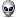# 藕山教育网 >>全册教案>>课例分析

## 一年级下册第二单元“20以内退位减法”练习检测题

 一、 填空题 1．计数器上，从右边数起 ，第一位是（    ）位，第二位是（    ）位，第三位是（    ）位。 2．5个十是（    ），（    ）个十是100。   3．7个十和8个一合起来是（    ），   4、6个一和8个十合起来是（    ）。   5．92里面有（    ）个一和（    ）个十，（   ）个一和（   ）个十组成32。   6．74是 （    ）位数，74里有（     ）个十和（   ）个一。   7．一个数，个位上3，十位上8，这个数是（    ），读作（            ）。   8．57里，5在（  ）位上，表示5个（  ），7在（   ）位上，表示7个（    ）。   9．88左边的8在（    ）位，表示（  ）个（    ），右边的8在（   ）位，表示（    ）个（      ）   10．69前面的一个数是（   ），后面的一个数是（   ）。和99相邻的两个数是（   ）和（   ）。   11．最大的两位数是（   ），最小的两位数是（   ），它们的差是（   ）。   12．比89大1的数是（    ），比它小1的数是（   ）。   13．60比（  ）大1，比（   ）小1。   14．一个数个位上是5，十位上的数比个位大1，这个数是(    )。   15．你最喜欢的十位上是6的两位数有(    )(    )(    )。   16．你最喜欢的个位上是6的两位数有(    )(    )(    )。   17．比45大，比60小的两位数中，个位是9的数有（   ）、（    ）。     二. 在○里填“>”、“<”、“=”．   　5○11-5     　7○12-5       14-6○9     　11-2○4   7+4○7+5      8+8○7+9　 12-6○14-7　 13-6○14-6   2. 　　15-( 　 )=7　　　 4+( 　 )=11　　　 11-( 　 )=5   　　　 ( 　 )-9=3    　　9+( 　 )=14　　　 12-( 　 )=8   　　　 7+( 　 )=12　　 13-( 　 )=6    　　( 　 )+6=11   　　　 13-( 　 )=4　　 ( 　 )+8=15　　　 ( 　 )-6=6   三、 口算题   1.　　13-6=　　 　8+7=　　　11-3=　　 　14-5=   　 　6+9=　　 　15-7=　　　 17-8=　　　 14-7=   　 　9+6=　　　 11-7=        12-7=　　 　3+8=   　　 14-6=　　 　13-5=　　 　4+8=　　 　12-9=   　 　8+6=　　 　16-7=　　 　13-4=　　 　11-9=       　12-8=      13-7=   　  11-6=        13-9=   9+7=       14-3=      18-7=        16-2=   15-6=　   　6+7=　　   12-3=　　     9+4=　　   　6+5=　   　 9+9=　　   6+6=　　 　 7+8=　　   　　 18-9=　　   12-6=　　    8+7=          14-9=   四、 文字叙述题   1. 一个加数是7, 另一个加数是9, 和是多少?   2. 被减数是12, 减数是4, 差是多少?   五、 应用题   1. 飞机场上有11架飞机, 又飞来5架, 现在有多少架?   2. 盒子里的小皮球取出9个, 还剩下7个, 盒子里原来有几个小皮球?   3. 张生种了6棵树, 加上秋生种的一共15棵, 秋生种了多少棵?   4. 大生有13个气球, 里面有8个红气球, 其余的是黄气球, 黄气球有多少个? (责任编辑：风)

(0)
0%

(0)
0%
------分隔线----------------------------

••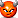•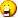•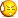•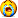•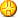•